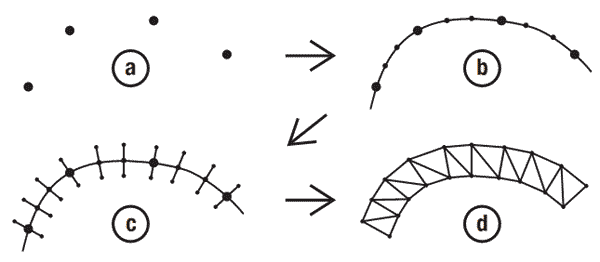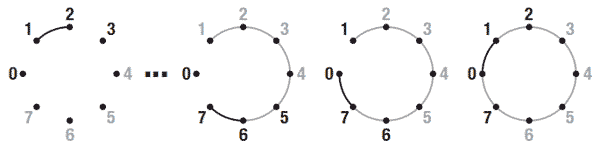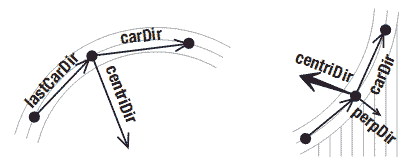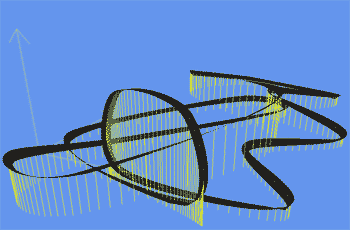# 5.17 为赛道创建顶点

## 解决方案## 工作原理

List<Vector3> trackPoints = new List<Vector3>();
trackPoints.Add(new Vector3(-2, 0, 4)); 

### 计算基点之间的额外点private List<Vector3> GenerateTrackPoints(List<Vector3> basePoints)
{

List<Vector3> allPoints = new List<Vector3>();
for (int i = 1; i < basePoints.Count-2; i++)
{
List<Vector3> part = InterpolateCR(basePoints[i - 1], basePoints[i], basePoints[i + 1], basePoints[i + 2]);
}

return allPoints; } 

for循环会继续直到所有部分的所有额外点都被添加到allPoints集合中。

### 计算外部的side点

Vector3 carDir = basePoints[i + 1] - basePoints[i];
Vector3 sideDir =Vector3.Cross(new Vector3(0, 1, 0), carDir);
sideDir.Normalize();
Vector3 outerPoint = basePoints[i] + sideDir * halfTrackWidth;
Vector3 innerPoint = basePoints[i] - sideDir * halfTrackWidth; 

### 创建顶点

VertexPositionNormalTexture vertex;
vertex = new VertexPositionNormalTexture(innerPoint, new Vector3(0, 1, 0), new Vector2(0, distance / textureLength));

vertex = new VertexPositionNormalTexture(outerPoint, new Vector3(0, 1, 0), new Vector2(1, distance / textureLength));

distance += carDir.Length(); 

private VertexPositionNormalTexture[] GenerateTrackVertices(List<Vector3> basePoints)
{
float halfTrackWidth = 0.2f;
float textureLength = 0.5f;
float distance = 0;
List<VertexPositionNormalTexture> verticesList = new List<VertexPositionNormalTexture>();

for (int i = 1; i < basePoints.Count-1; i++)
{
Vector3 carDir = basePoints[i + 1] - basePoints[i];
Vector3 sideDir = Vector3.Cross(new Vector3(0, 1, 0), carDir);
sideDir.Normalize();
Vector3 outerPoint = basePoints[i] + sideDir * halfTrackWidth;
Vector3 innerPoint = basePoints[i] - sideDir * halfTrackWidth;

VertexPositionNormalTexture vertex;
vertex = new VertexPositionNormalTexture(innerPoint, new Vector3(0, 1, 0), new Vector2(0, distance / textureLength));

vertex = new VertexPositionNormalTexture(outerPoint, new Vector3(0, 1, 0), new Vector2(1, distance / textureLength));
}

VertexPositionNormalTexture extraVert = verticesList;
extraVert.TextureCoordinate.Y = distance / textureLength;

extraVert = verticesList;
extraVert.TextureCoordinate.Y = distance / textureLength;

return verticesList.ToArray();
}

for循环之后，verticesList包含了赛道每个中心点的两个顶点。但是，当你从顶点集合绘制三角形时，仍会在最后一个中心点和第一个中心点之间出现缝隙。要连接这个缝隙，你需要将前两个中心点的side点复制到集合中。但是，因为前两个顶点的Y坐标为0，你需要将它们调整到当前的纹理坐标值。否则，最后两个三角形将会将它们的Y坐标回到0，导致大量的纹理堆砌在两个小三角形上。

### 绘制赛道

List<Vector3> extendedTrackPoints = GenerateTrackPoints(basePoints);
trackVertices = GenerateTrackVertices(extendedTrackPoints); 

basicEffect.World = Matrix.Identity;
basicEffect.View = fpsCam.ViewMatrix;
basicEffect.Projection = fpsCam.ProjectionMatrix;
basicEffect.TextureEnabled = true;
basicEffect.VertexColorEnabled = false;

basicEffect.Begin();
foreach (EffectPass pass in basicEffect.CurrentTechnique.Passes)
{
pass.Begin();
device.VertexDeclaration = myVertexDeclaration;
device.DrawUserPrimitives<VertexPositionNormalTexture>(PrimitiveType.TriangleStrip,trackVertices, 0, trackVertices.Length - 2);
pass.End();
}
basicEffect.End(); 

### 围栏

Vector3 currentNormal = Vector3.Up;Vector3 carDir = trackPoints[i + 1] - trackPoints[i];
carDir.Normalize();
Vector3 lastCarDir = trackPoints[i] - trackPoints[i - 1];
lastCarDir.Normalize();
Vector3 perpDir = Vector3.Cross(carDir, lastCarDir);
Vector3 centriDir = Vector3.Cross(carDir, perpDir); 

currentNormal = currentNormal + centriDir * banking + Vector3.Up/banking;
currentNormal.Normalize(); 

banking变量的值越大， 赛道添加的围栏越多。这里起你可以使用前面的代码，但别忘了用currentNormal代替(0,1,0) Up向量：

Vector3 sideDir = Vector3.Cross(currentNormal, carDir);
sideDir.Normalize();
currentNormal = Vector3.Cross(carDir, sideDir);

## 代码

GenerateTrackPoints方法接受基点的数组并进行了扩展，让这个方法可以添加赛道的细节：

private List<Vector3> GenerateTrackPoints(List<Vector3> basePoints)
{

List<Vector3> allPoints = new List<Vector3>();
for (int i = 1; i < basePoints.Count-2; i++)
{
List<Vector3> part = InterpolateCR(basePoints[i - 1], basePoints[i], basePoints[i + 1], basePoints[i + 2]);
}
return allPoints;
}

private VertexPositionNormalTexture[] GenerateTrackVertices(List<Vector3>trackPoints)
{
float halfTrackWidth = 0.2f;
float textureLength = 0.5f;
float banking = 2.0f;
float distance = 0;
List<VertexPositionNormalTexture> verticesList = new List<VertexPositionNormalTexture>();
Vector3 currentNormal = Vector3.Up;

for (int i = 1; i < trackPoints.Count-1; i++)
{
Vector3 carDir = trackPoints[i + 1] - trackPoints[i];
carDir.Normalize();
Vector3 lastCarDir = trackPoints[i] - trackPoints[i - 1];
lastCarDir.Normalize();
Vector3 perpDir =Vector3.Cross(carDir, lastCarDir);
Vector3 centriDir = Vector3.Cross(carDir,perpDir);

currentNormal = currentNormal + Vector3.Up/banking + centriDir * banking;
currentNormal.Normalize();
Vector3 sideDir = Vector3.Cross(currentNormal, carDir);
sideDir.Normalize();
currentNormal = Vector3.Cross(carDir, sideDir);

Vector3 outerPoint = trackPoints[i] + sideDir * halfTrackWidth;
Vector3 innerPoint = trackPoints[i] - sideDir * halfTrackWidth;
distance += carDir.Length();

VertexPositionNormalTexture vertex;
vertex = new VertexPositionNormalTexture(innerPoint, currentNormal, new Vector2(0, distance / textureLength));
vertex = new VertexPositionNormalTexture(outerPoint, currentNormal, new Vector2(1, distance / textureLength));
}

VertexPositionNormalTexture extraVert = verticesList;
extraVert.TextureCoordinate.Y = distance / textureLength;
extraVert = verticesList;
extraVert.TextureCoordinate.Y = distance / textureLength;

return verticesList.ToArray();
}

List<Vector3> basePoints = new List<Vector3>();
trackVertices = GenerateTrackVertices(extendedTrackPoints);